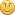## A function only Landscape : Part Four - Putting it all together.

Started by David Burnett, March 25, 2007, 02:33:34 pm

#### David Burnett

#####March 25, 2007, 02:33:34 pm
To create my function only landscape I'm going to use the pyramid, the linear sand dunes and Perlin's noise.

To to start off create a linear sand dune node network and add a pyramid node network, or load the tutorial4_start tgd file.

[attachurl=1]

Now we will put some kinks in those straight lines, using Perlin's noise.

• Add a Perlin 3D scalar, a  Constant Scalar, a Multiply Scalar and an Add Scalar node.

• Connect the linear dunes Get Position to the Perlin 3D scalar.

• Connect the output of Perlin 3D scalar and the Constant Scalar to the Multiply node .

• Connect the Multiply Scalar node to the Add Node.

• To distort the Noise connect the X to Scalar node to the new Add node, and feed the Add

• node into the linear dunes Modulo node instead of the X to Scalar Node.

• Set the New Constant node to 1 to start of with and play with the value until you like

• the distortion. I'm going to use 1.0.

To add the pyramid into the scene we need to use our old friend the Condition Scalar.
Were the pyramid overlaps the sand dunes we want to pick the highest value, so the
condition we want is "Greater than".

• Add a Conditional Scalar, set the Condition to "Greater Than".

• Connect the end of linear dune node network to the Input Node and If result.

• Connect the pyramid node network to Comparison value and Else result.

• Connect the Conditional Scalar output to the Displacement Shader.

You may have to look around for the pyramid or you could just set the camera to position 395, 15, -800 and rotation -0.5,-35, 0

It's a bit flat so lets add some big dunes, using Perlin's noise again.

• Add a  Perlin 3D scalar, two Constant Scalars, a Multiply Scalar and an Add Scalar node.

• Connect a Get Position to the Perlin 3D scalar.

• Connect a Constant Scalar to the Scale input node of the Perlin 3D scalar, Set the constant to 25.0 .

• Connect the output of Perlin 3D scalar and the other Constant Scalar, set to 10.0, to the Multiply node .

This time we do not want the Highest value to use used as we want linear dunes on the other dunes
so to do this we add the new Perlin noise on the linear dunes.

• Connect the Multiply Scalar node to the Add Node.

• Connect the Conditional Scalar at the end of the linear sand dune node network to the new Add Node.

Now we need to tie the the dunes into the Conditional Scalar before the Displacement shader, however I'm
going to do it in a Surprisingly different way.

• Connect the new Add Node to the If result input on the Conditional Shader.

I've left linear dune node network feeding into the Input Node. This means that where the linear dunes
were visible before the new dunes will be used. All we need to do now is change the Base Color Shader
to something that looks Sandy and hopefully you have something that looks like this..

[attachimg=2]

Here's the finished tgd in case you got lost

[attachurl=3]

You might want to connect the dunes to the Input Node to see the effect, and for extra marks work how to make the plains
it creates use the linear dunes.

#### Oshyan

#####March 25, 2007, 03:06:54 pm #1
Ah, here we go, the rendered result.As I said elsewhere linking to the relevant discussion thread(s) could be good too. Great work!